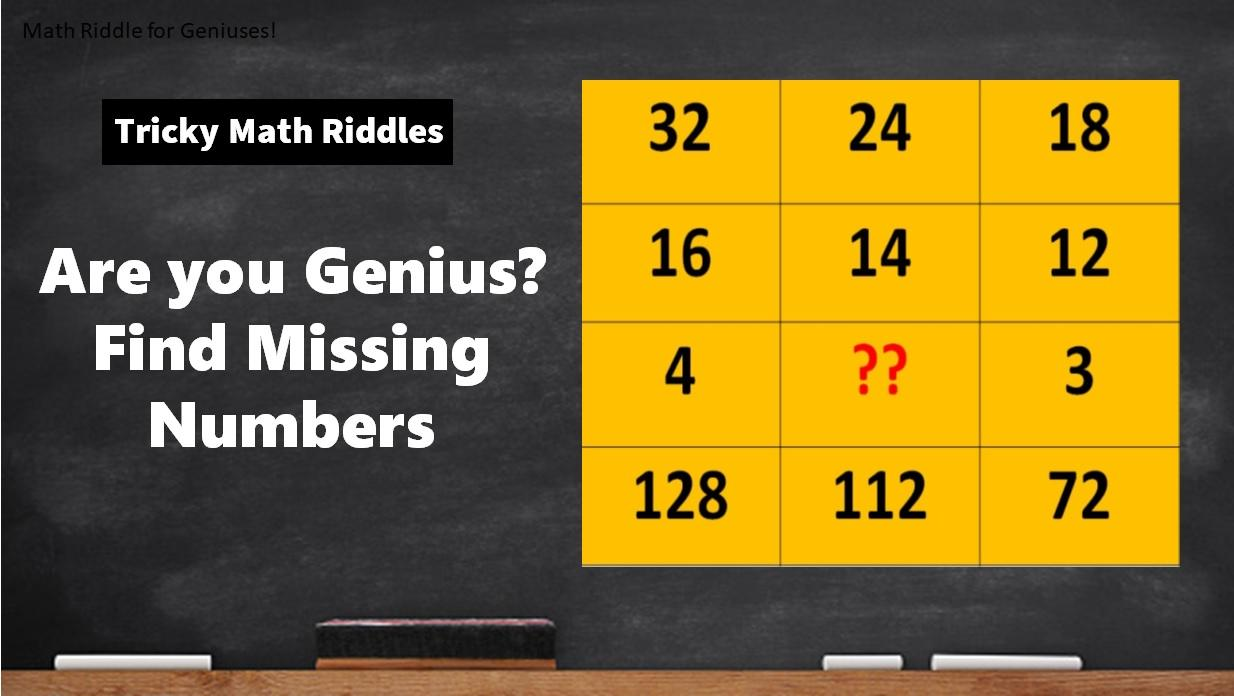# Math Riddles with Answers: Are you Genius? Find All Missing Numbers

Math Riddles with Answers: Can you find the missing numbers in these tricky math puzzles? Only 1% of people with a high IQ can get the answer right. Check out these math riddles for geniuses!Math Riddles with Answers: Are you Genius? Find All Missing Numbers

Math Riddles are so challenging, but that makes them worthwhile to solve. Math riddles are logical problems that require strong analytical abilities, high IQ, knowledge of math concepts, and good calculation skills. Math riddles have also been known to make Mathematics enjoyable for kids, students, and even adults to solve. Riddles, puzzles, and brain teasers can develop strategic thinking, logical reasoning, and problem-solving skills.

Can you solve all the math puzzles? You have 20 seconds for each riddle!

## Math Riddle #5

Also Read: Math Riddles with Answers: Only the 1% Smartest Can Find All Missing Numbers

Math Riddle #1

You have to divide 30 by half, not by 2.

So, the equation will be

= 30 x 2 + 12 = 72

Math Riddle #2

1 + 9 = 10

1 x 9 = 9

Math Riddle #3

1 + 2 + 3 = 6

1 x 2 x 3 = 6

Math Riddle #4

444 + 44 + 4 + 4 + 4 = 500

Math Riddle #5

 Column #1 Column #2 Column #3 Row #1 32 24 18 Row #2 16 14 12 Row #3 4 ?? 3 Row #4 128 112 72

To solve this puzzle, we first understand that:

For Column #1: row #4 ÷ row #2 = quotient and quotient x row #3 = row #1

128  ÷ 16 = 8 and 8 x 4 = 32

For Column 3: row #4 ÷ row #2 = quotient and quotient x row #3 = row #1

72  ÷ 12 = 6 and 6 x 3 = 18

Similarly, for Column 2, we will find the missing number with the same equation:

row #4 ÷ row #2 = quotient and quotient x row #3 = row #1

112  ÷ 14 = 8 and 8 x ?? = 24 (so we know that to get the product as in row #1, we need to multiply the quotient by 3 which will equal 24.

## Tell us in comments: Did you solve all these tricky math riddles?

Also Read: Math Riddles: Only High IQ Genius Can Solve These

Also Read: Tricky IQ Riddles: Only 1 in 5 people with high IQ can solve these

Also Read:  What Am I? Riddles: Only Smartest 1% Can Solve These Tricky Puzzles

Also Read: World’s Toughest Riddle: Only High IQ Genius Can Solve This Math Riddle